Get instant live expert help with Excel or Google Sheets“My Excelchat expert helped me in less than 20 minutes, saving me what would have been 5 hours of work!”

#### Post your problem and you’ll get expert help in seconds.

Your message must be at least 40 characters
Our professional experts are available now. Your privacy is guaranteed.

# Round a number down

We use the ROUNDDOWN function to round a number down, not minding the number being rounded to a specified number of decimal places. We will walk through the process of using the ROUNDDOWN function in simple steps.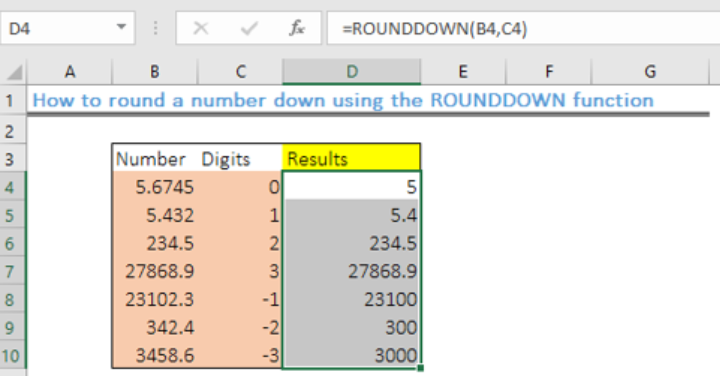Figure 1- Results of using the ROUNDDOWN function

## General Formula

`=ROUNDDOWN(number,num_digits)`

• “Number” – number to round down
• “num_digits – the number of decimal places to round down the number.

## Formula

`=ROUNDDOWN(B4,C4)`

## Setting up the data

• We will input the numbers to be rounded up in the range: B4:B10
• Next, we specify the number of digits in the range C4:C10
• We will place the results in Column D, which we name “Results.”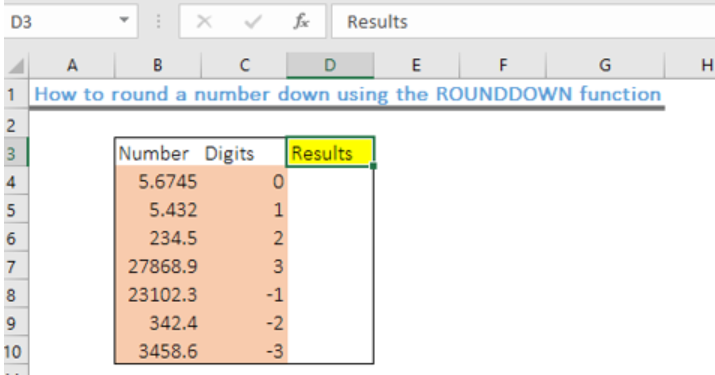Figure 2 – Setting up the Data

## Applying the Excel ROUNDDOWN function

• We will click on Cell D4
• We will insert the formula below in Cell D4

`=ROUNDDOWN(B4,C4)`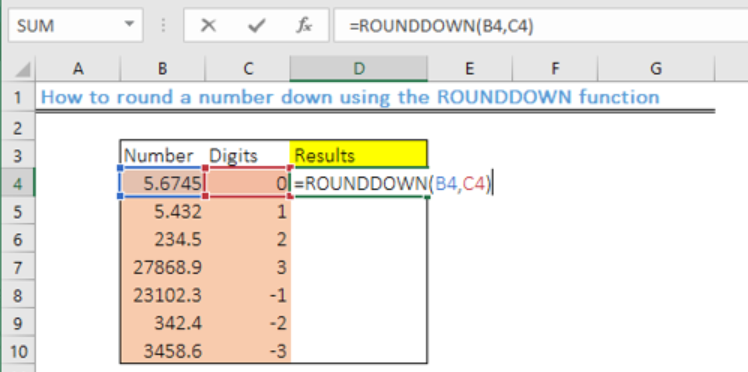Figure 3 – Applying the ROUNDDOWN function

• We will press the enter key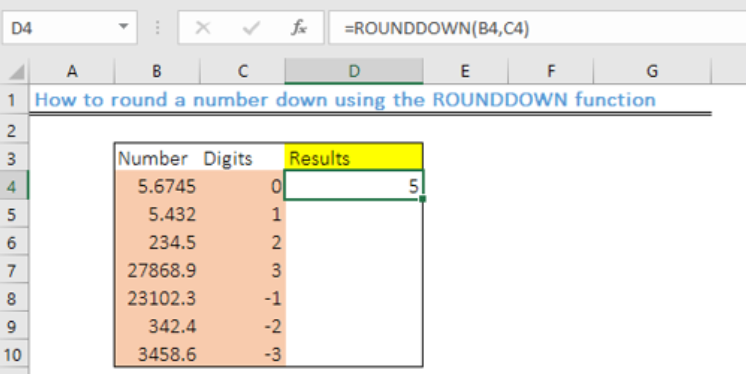Figure 4- Cell containing a rounded down number

• We will use the fill handle tool to copy the formula into the other cells to get the results.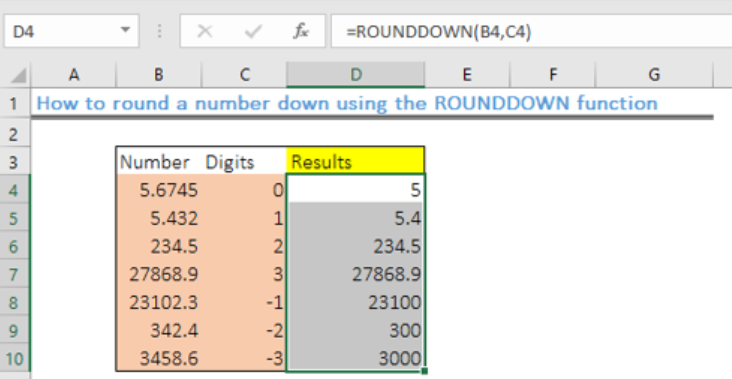Figure 5-How to use the Excel ROUNDDOWN function

## Explanation

The ROUNDDOWN function will always round numbers 1-9 down. This is unlike the standard rounding that rounds numbers less than 5 down. It works like the Excel ROUND function and positive digits will be rounded to the right of the decimal point while zero and negative numbers will be rounded to the left.

Therefore:

• If num_digits is > 0, the number will be rounded to the right of the decimal point
• If num_digits is < 0, the number is rounded down to the left of the decimal.
• If num_digits = 0, the number is rounded down to the left of the decimal point

## Instant Connection to an Expert through our Excelchat Service

Most of the time, the problem you will need to solve will be more complex than a simple application of a formula or function. If you want to save hours of research and frustration, try our live Excelchat service! Our Excel Experts are available 24/7 to answer any Excel question you may have. We guarantee a connection within 30 seconds and a customized solution within 20 minutes.

### Did this post not answer your question? Get a solution from connecting with the expert.Another blog reader asked this question today on Excelchat:
Solution examplesHow to calculate the average number of customers during different time periods
Solved by S. J. in 17 minsI have an IF statement that works. Its pretty complicated with VLOOKUP. But i need to make this an OR statement and i keep getting errors and can't figure out the formula.
Solved by F. S. in 30 mins## Subscribe to Excelchat.coAnother blog reader asked this question today on Excelchat: